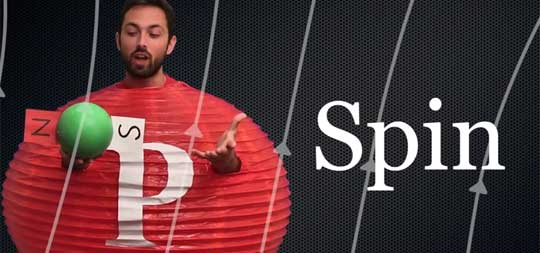How Does a Quantum Computer Work?A quantum computer works in a totally different way from a classical computer. Quantum bits or ‘qubits’ can exist in a superposition state of both zero and one simultaneously. This means that a set of two qubits can be in a superposition of four states, which therefore require four numbers to uniquely identify the state. So the amount of information stored in N qubits is two to the power of N classical bits.